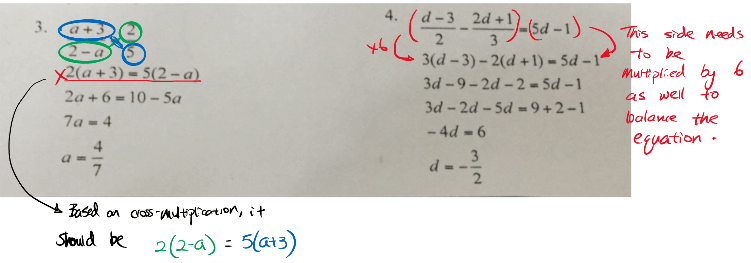# Question

Question 3:

The mistake appears in the first step.

(a+3)/(2-a) = 2/5 does not imply 2(a+3) = 5(2-a). That would involve a wrong transformation of the equation. The correct method is as such:

5 * (a + 3) / (2 – a) = 5 * 2/5 = 2

(2 – a) * 5 * (a + 3) / (2 – a) = (2 – a) * 2

5 (a + 3) = 2 (2 – a). And then, you solve for the equation as per normal.

Question 4:

Firstly, 9 + 2 – 1 = 10 not 6.

Secondly, in the first step, we are trying to remove the denominator by multiplying by 6 on both sides. Hence, we should get

3(d-3) – 2(2d-1) = 6(5d-1), rather than what’s shown in the question

0 Replies 0 Likes0 Replies 0 Likes